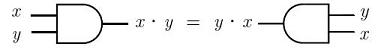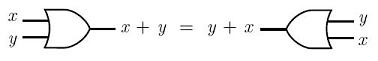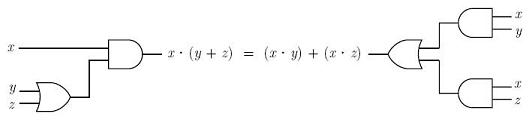# Axioms - Laws of Boolean Algebra

Boolean algebra is the algebra of propositions. Propositions are denoted by letters, such as A, B, x or y, etc.

 In the following axioms and theorems (laws of boolean algebra), the '+' or 'V' signs represent a logical OR (or conjunction), the '.' or '^' signs represent a logical AND (or disjunction), and '¬' or '~' represent a logical NOT ( or negation). Every proposition has two possible values: 1 (or T) when the proposition is true and 0 (or F) when the proposition is false.

The negation of A is written as ¬A (or ~A) and read as 'not A'. If A is true then ¬A is false. Conversely, if A is false then ¬A is true.

### Basic Laws of Boolean Algebra

 Descript. OR form AND form Other way to express it: Axiom x+0 = x x.1 = x A V F = A A ^ T = A Commutative x+y = y+x x.y = y.x A V B = B V A A ^ B = B ^ A Distributive x.(y+z) = (x.y)+(x.z) x+y.z = (x+y).(x+z) A ^ (B V C) = (A ^ B) V (A ^ C) A V B ^ C = (A V B) ^ (A V C) Axiom x+¬x = 1 x.¬x = 0 A V ¬A = T A ^ ¬A = F Theorem x+x = x x.x = x A V A = A A ^ A = A Theorem x+1 = 1 x.0 = 0 A V T = T A ^ F = F Theorem ¬¬x = x ¬(¬A) = A Associativity x+(y+z) = (x+y)+z x.(y.z) = (x.y).z A V (B V C) = (A V B) V C A ^ (B ^ C) = (A ^ B) ^ C Absorption x+x.y = x x.(x+y) = x A V A ^ B = A A ^ (A V B) = A DeMorgan's laws x+y = ¬(¬x.¬y) x.y = ¬(¬x+¬y) A V B = ¬(¬A ^ ¬B) A ^ B = ¬(¬A V ¬B)

Using logical gates, the commutative property for a logical AND is:The commutative property for a logical OR, is:Using electronic gates, the distributive property is:The De Morgan's laws can transform logical ORs into logical ANDs (negations are necessary) and can electronically be described this way:orFrom 'Axioms' to home

From 'Axioms' to 'Boolean Algebra'